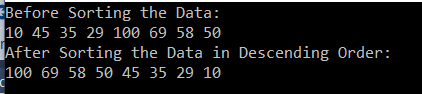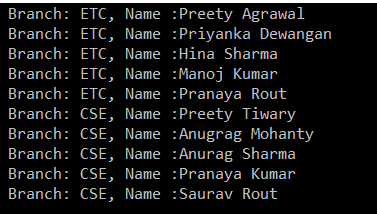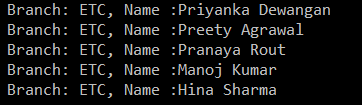# Linq OrderByDescending Method in C#

## LINQ OrderByDescending Method in C# with Examples

In this article, I am going to discuss the LINQ OrderByDescending Method in C# with examples. Please read our previous article where we discussed the OrderBy Method in C# with some examples. As part of this article, we are going to discuss the following pointers related to the  LINQ OrderByDescending method.

1. What is Linq OrderByDescending Method in C#?
2. Example of Linq OrderByDescending Method using both Method and Query Syntax.
3. How to use Linq OrderByDescending Method with Complex Type in C#?
4. How to use the OrderByDescending method along with the Filtering method?

#### What is Linq OrderByDescending Method in C#?

The LINQ OrderByDescending method in C# is used to sort the data in descending order. The point that you need to remember is, the OrderByDescending method is not going to change the data, it is just changing the order of the data.

Like the OrderBy method, you can also use the OrderByDescending method on any data type such as string, character, float, integer, etc. Let us understand how to use the OrderByDescending method in C# using both query and method syntax.

##### Working with integer data

In the following example, we have an integer collection. And we need to sort the data in descending order. Let us see how to do this using both query and method syntax.

```using System;
using System.Collections.Generic;
using System.Linq;
namespace LINQDemo
{
class Program
{
static void Main(string[] args)
{
List<int> intList = new List<int>() { 10, 45, 35, 29, 100, 69, 58, 50 };

Console.WriteLine("Before Sorting the Data: ");
foreach (var item in intList)
{
Console.Write(item + " ");
}

//Sorting the data in Descending Order
//Using Method Syntax
var MS = intList.OrderByDescending(num => num);

//Using Query Syntax
var QS = (from num in intList
orderby num descending
select num).ToList();

Console.WriteLine();
Console.WriteLine("After Sorting the Data in Descending Order: ");
foreach (var item in QS)
{
Console.Write(item + " ");
}

}
}
}```

Output:##### Working with string data.

In the following example, we have a collection of string data i.e. a collection of names. We want to sort the data in descending order using both method and query syntax. Let us see how we can do this.

```using System;
using System.Collections.Generic;
using System.Linq;
namespace LINQDemo
{
class Program
{
static void Main(string[] args)
{
List<string> stringList = new List<string>() { "Preety", "Tiwary", "Agrawal", "Priyanka", "Dewangan",
"Hina","Kumar","Manoj", "Rout", "James"};

//Using Method Syntax
var MS = stringList.OrderByDescending(name => name);

//Using Query Syntax
var QS = (from name in stringList
orderby name descending
select name).ToList();

foreach (var item in MS)
{
Console.WriteLine(item + " ");
}

}
}
}```
##### LINQ OrderByDescending Method with Complex type in C#:

In order to understand how to work with complex type, we are going to work with the following Student class. So, create a class file with the name Student.cs and then copy and paste the following code in it.

```using System.Collections.Generic;
namespace LINQDemo
{
public class Student
{
public int ID { get; set; }
public string FirstName { get; set; }
public string LastName { get; set; }
public string Branch { get; set; }

public static List<Student> GetAllStudents()
{
List<Student> listStudents = new List<Student>()
{
new Student{ID= 101,FirstName = "Preety",LastName = "Tiwary",Branch = "CSE"},
new Student{ID= 102,FirstName = "Preety",LastName = "Agrawal",Branch = "ETC"},
new Student{ID= 103,FirstName = "Priyanka",LastName = "Dewangan",Branch = "ETC"},
new Student{ID= 104,FirstName = "Hina",LastName = "Sharma",Branch = "ETC"},
new Student{ID= 105,FirstName = "Anugrag",LastName = "Mohanty",Branch = "CSE"},
new Student{ID= 106,FirstName = "Anurag",LastName = "Sharma",Branch = "CSE"},
new Student{ID= 107,FirstName = "Pranaya",LastName = "Kumar",Branch = "CSE"},
new Student{ID= 108,FirstName = "Manoj",LastName = "Kumar",Branch = "ETC"},
new Student{ID= 109,FirstName = "Pranaya",LastName = "Rout",Branch = "ETC"},
new Student{ID= 110,FirstName = "Saurav",LastName = "Rout",Branch = "CSE"}
};

return listStudents;
}
}
}```

As you can see, we created the above Student class with four simple properties (ID, FirstName, LastName, and Brach). We then created one method (i.e. GetAllStudents) which will return the list of all students.

##### Sorting the Data in Descending Order

Here, we want to sort the data based on the Branch in descending order.

```using System.Linq;
namespace LINQDemo
{
class Program
{
static void Main(string[] args)
{
//Method Syntax
var MS = Student.GetAllStudents().OrderByDescending(x => x.Branch).ToList();

//Query Syntax
var QS = (from std in Student.GetAllStudents()
orderby std.Branch descending
select std);

foreach (var student in MS)
{
Console.WriteLine(" Branch: " + student.Branch + ", Name :" + student.FirstName + " " + student.LastName);
}

}
}
}```

Output:##### Linq OrderByDescending with Filtering Operator.

Now we need to fetch only the ETC branch students and then we need to sort the students based on their FirstName in descending order.

Note: The most important point that you need to keep in mind is, you need to use the Where extension method before the OrderByDescending method.

```using System.Linq;
namespace LINQDemo
{
class Program
{
static void Main(string[] args)
{
//Method Syntax
var MS = Student.GetAllStudents()
.Where(std => std.Branch.ToUpper() == "ETC")
.OrderByDescending(x => x.FirstName).ToList();

//Query Syntax
var QS = (from std in Student.GetAllStudents()
where std.Branch.ToUpper() == "ETC"
orderby std.FirstName descending
select std);

foreach (var student in QS)
{
Console.WriteLine(" Branch: " + student.Branch + ", Name :" + student.FirstName + " " + student.LastName);
}